# Surface and Volume Equations

Cylinder
Sphere
Hollow Cylinder
Cone
Torus
Spherical Sector
Cylinder Portion
Cylinder

 Equations Configuration Where: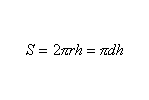Equation for calculating cylinder surface area Cylinder Surface r is the radius d is the diameter h is the height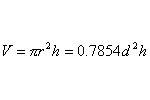Equation for calculating cylinder volume Cylinder Volume r is the radius d is the diameter h is the height

Sphere

 Equations Configuration Where:Equation for calculating sphere surface area Sphere Surface r is the radius d is the diameterEquation for calculating sphere volume Sphere Volume r is the radius d is the diameter

Hollow Cylinder

 Equations Configuration Where: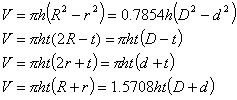Equation for calculating hollow cylinder volume Hollow Cylinder Volume R is the outer radius r is the inner radius D is the outer diameter d is the inner diameter h is the height t is the thickness

Cone

 Equations Configuration Where:Equation for calculating cone surface area Cone Surface r is the base radius h is the height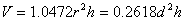Equation for calculating cone volume Cone Volume r is the base radius d is the base diameter h is the height

Torus

 Equations Configuration Where:Equation for calculating torus surface area Torus Surface R is the mean radius r is the minor radius D is the mean diameter d is the minor diameter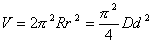Equation for calculating torus volume Torus Volume R is the mean radius r is the minor radius D is the mean diameter d is the minor diameter

Spherical Sector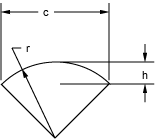Diagram for calculating spherical sector surface area and volume

 Equations Configuration Where: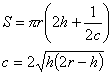Equation for calculating spherical sector surface area Spherical Sector Surface r is the radius h is the height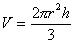Equation for calculating spherical sector volume Spherical Sector Volume r is the radius h is the height

Cylinder Portion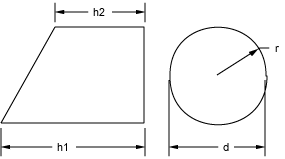Diagram for calculating cylinder portion surface area and volume

 Equations Configuration Where: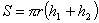Equation for calculating cylinder portion surface area Cylinder Portion Surface r is the radius h1 is the 1st height h2 is the 2nd height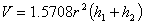Equation for calculating cylinder portion volume Cylinder Portion Volume r is the radius h1 is the 1st height h2 is the 2nd height